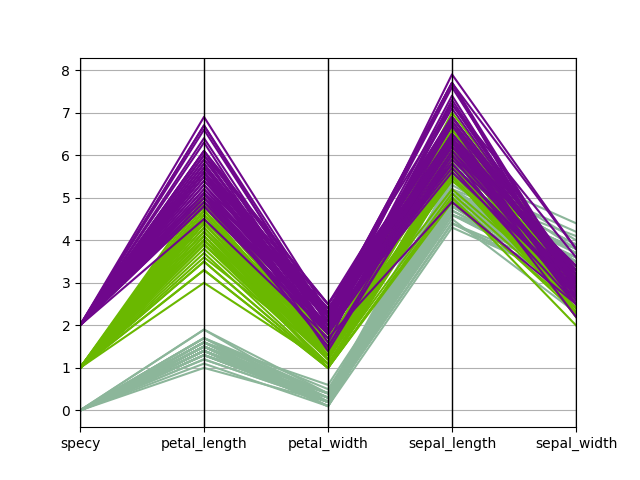# Parallel coordinates¶

from __future__ import annotations

from gemseo.api import configure_logger
from gemseo.post.dataset.parallel_coordinates import ParallelCoordinates

configure_logger()

<RootLogger root (INFO)>


iris = load_dataset("IrisDataset")


### Plot parallel coordinates¶

We can use the ParallelCoordinates plot, a.k.a. cowebplot, where each samples is represented by a continuous straight line in pieces whose nodes are indexed by the variables names and measure the variables values.

ParallelCoordinates(iris, classifier="specy").execute(save=False, show=True)[<Figure size 640x480 with 1 Axes>]


Total running time of the script: ( 0 minutes 0.293 seconds)

Gallery generated by Sphinx-Gallery